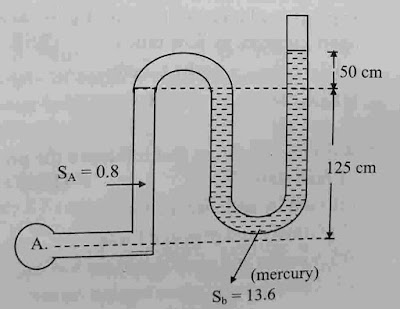Mcq on manometer (objective questions)

Hello guys we know manometer are used to measure pressure of fluid.  In This lesson today we solved fluid mechanics topic multiple choice questions Mcq on manometer (objective questions)

Guys this manometer mcq is important for all competitive exam.

Known without wasting time let's begin to start manometer mcq (objectives) questions with answers.

Mcq on manometer (objective questions)Mcq on manometer (objective questions)

Know start mcq

1. Pressure of fluid is measured by a .............. instrument.

A. Thermometer

B. Lactometer

C. Manometer.

D. Barometer

Ans - C

2. A manometer is used to measure...........

A. Low pressure

B. High pressure

C. Moderate pressure

D. Atmospheric pressure

Ans - B

3. A manometer is used to measure.............

A. Pressure in venturimeter

B. Atmospheric pressure

C. Pressure in pipes,channel

D. Difference pressure between two point

Ans - C

4. Liquid used in manometer should have.......

A. High density

B. Low density

C. High surface tension

D. Low surface tension

Ans - A

5. Micromanometer is used to measure.......

A. High pressure difference

B. Low pressure difference

C. Pressure measured for liquid only.

D. Pressure measured for gas only.

Ans - B

6. Manometer can be used to measure vaccum pressure (negative pressure)

A. True

B. False

Ans - A

Solution

Manometer is measure vaccum pressure and gauge pressure.

7. A deferential manometer generally measure of .........

A. Pressure in pipe

B. Atmospheric pressure

C. Pressure in channel

D. Measure difference pressure between two points

Ans - D

8. A monometric liquid should suitable have...........

A. Low vapour pressure and high density

B. High vapour pressure and high density

C. Low vapour pressure and low density

D. High vapour pressure and high density

Ans - A

9. Which of the following is not pressure sensing elements?

A. Burdon tube

B. Bellows

C. Manometer

D. Orifice plate

Ans - D

10. Simple U tube manometer can measure both positive gauge pressure and negative (vaccum) gauge pressure.

A. True

B. False

Ans - A

11. Inclined manometer are used for measuring of low pressure

A. True

B. False

Ans - A

12. Manometer is device used for measuring of..........

A. Discharge of a fluid

B. Velocity of fluid

C. Pressure at a fluid

D. None of a above these

Ans - C

13. Piezometer is manometer that used for measuring of.........

A. Positive gauge pressure

B. Negative gauge pressure

C. Both Positive and negative gauge pressure.

D. none of above these

Ans - A

14. Piezometer is manometer that used for measuring of.........

A. Low pressure

B. Moderate pressure

C. High pressure

D. none of these

Ans - B

15. U tube manometer measure the.........

A. Pressure in pipe

B. Atmospheric pressure

C. Pressure in channel

D. Measure difference pressure between two points

Ans - D

16. Show in figure of manometer. What is absolute pressure at point A.

(Assume atmospheric pressure 103 KN/m²)

(Where SA = specific gravity of A fluid

SB = specific gravity of B fluid)Manometer is used to measure

A. 175 KN/m²

B. 180 KN/m²

C. 190 kN /m²

D. 195 KN/m²

Ans - B

In This lesson today we solved fluid mechanics topic multiple choice questions Mcq on manometer (objective questions)

If you have any questions about manometer then comment below
Previous
Next Post »